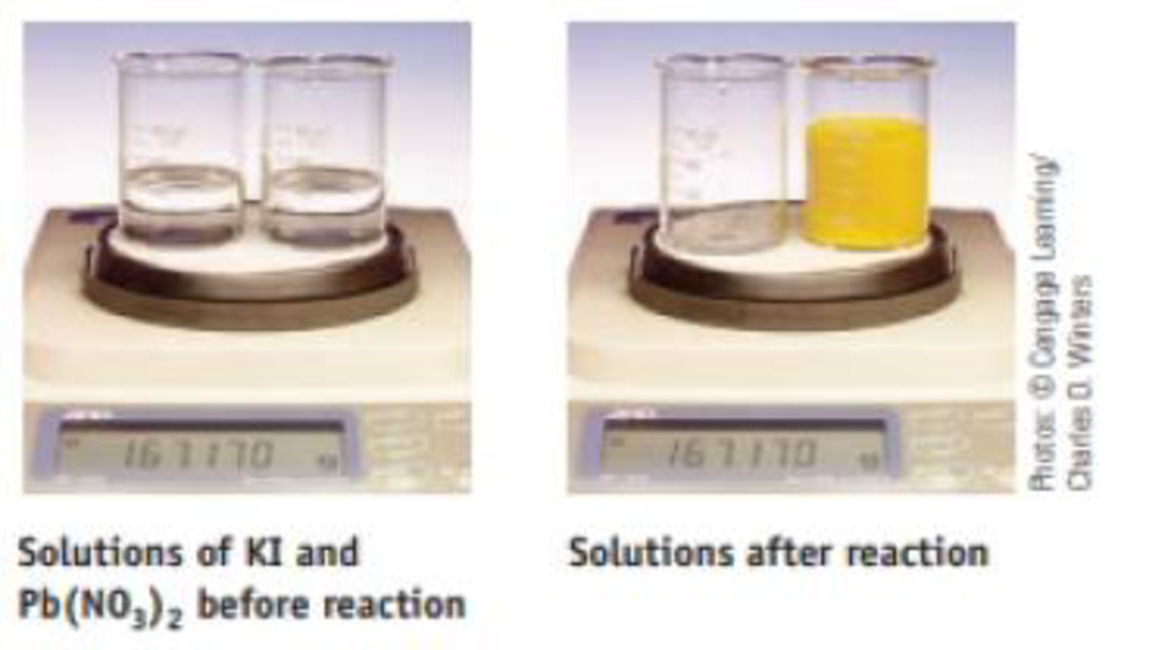# Two beakers sit on a balance; the total mass is 167.170 g. One beaker contains a solution of KI; the other contains a solution of Pb(NO 3 ) 2 . When the solution in one beaker is poured completely into the other, the following reaction occurs: 2 KI(aq) + Pb(NO 3 ) 2 (aq) → 2 KNO 3 (aq) + PbI 2 (s) What is the total mass of the beakers and solutions after reaction? Explain completely.### Chemistry & Chemical Reactivity

9th Edition
John C. Kotz + 3 others
Publisher: Cengage Learning
ISBN: 9781133949640

#### Solutions

Chapter
Section### Chemistry & Chemical Reactivity

9th Edition
John C. Kotz + 3 others
Publisher: Cengage Learning
ISBN: 9781133949640
Chapter 4, Problem 135SCQ
Textbook Problem
102 views

## Two beakers sit on a balance; the total mass is 167.170 g. One beaker contains a solution of KI; the other contains a solution of Pb(NO3)2. When the solution in one beaker is poured completely into the other, the following reaction occurs:2 KI(aq) + Pb(NO3)2(aq) → 2 KNO3(aq) + PbI2(s)What is the total mass of the beakers and solutions after reaction? Explain completely.

Interpretation Introduction

Interpretation:

The total mass of the beakers and solutions after the given reaction has to be determined and explained.

Concept introduction:

• For chemical reaction balanced chemical reaction equation written in accordance with the Law of conservation of mass.
• Law of conservation of mass states that for a reaction total mass of the reactant and product must be equal.

### Explanation of Solution

The balanced chemical reaction equation for the given reaction is,

2KI(aq)+Pb(NO3)2(aq)2KNO3<

### Still sussing out bartleby?

Check out a sample textbook solution.

See a sample solution

#### The Solution to Your Study Problems

Bartleby provides explanations to thousands of textbook problems written by our experts, many with advanced degrees!

Get Started

Find more solutions based on key concepts
Cells a. are self-contained, living units. b. serve the bodys needs but have few needs of their own. c. remain ...

Nutrition: Concepts and Controversies - Standalone book (MindTap Course List)

What is the maximum number of bonds that a carbon atom can form?

Biology: The Dynamic Science (MindTap Course List)

What are the starting products for photosynthesis? The end products?

Oceanography: An Invitation To Marine Science, Loose-leaf Versin

Rank the magnitudes of Bds for the closed paths a through d in Figure 29.11 from greatest to least. Figure 29.1...

Physics for Scientists and Engineers, Technology Update (No access codes included)